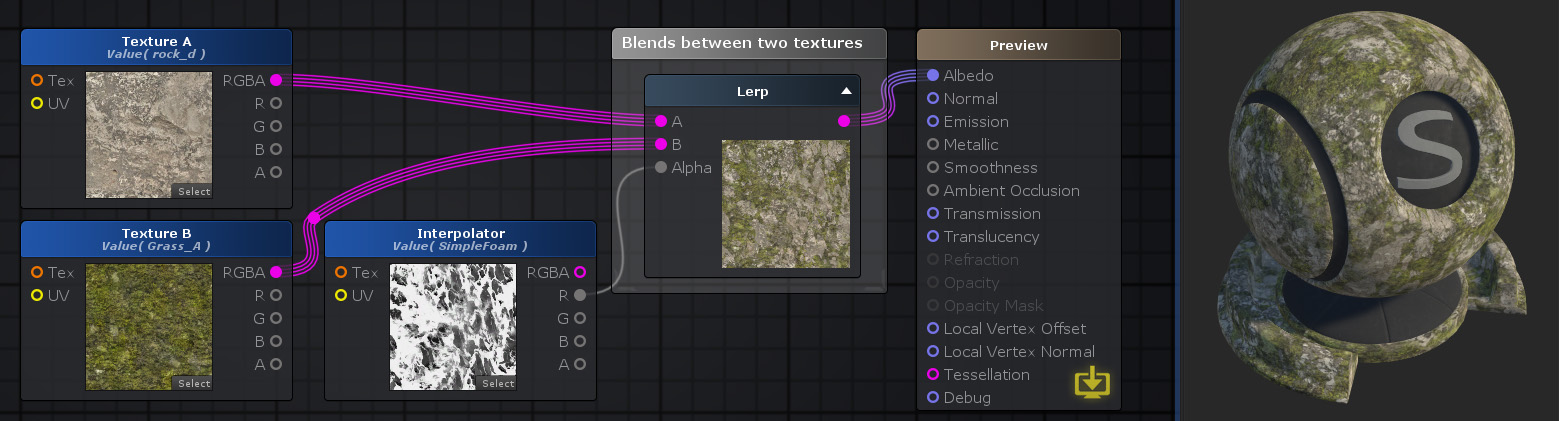# Unity Products:Amplify Shader Editor/Lerp

Jump to: navigation, search

## Lerp Node

The Lerp node ( shortcut: L key ) calculates a linear interpolation between two values A and B by Alpha. In other words it will generate a new blended value between A and B according to a third one called Alpha, a.k.a Interpolator, using the the expression ( ( 1 - A ) * I + B * I ). The interpolator value is ranged between [0 - 1] on which it will return A if I = 0 and B if I = 1.

NOTE: Linear interpolation between multi-channel data types are done per-channel. If A and B have different channel amounts a cast is done to match the one with the most amount of channels.Nodes used: Texture Sample, Lerp

Node Parameter Description Default Value
A First value of the interpolator operation, fully outputted when Alpha = 0. Only visible if the respective input port is not connected. 0
B Last value of the interpolator operation, fully outputted when Alpha = 1. Only visible if the respective input port is not connected. 0
Alpha Interpolator value. Only visible if the respective input port is not connected. 0

Input Port Description Type
A First value of the interpolator operation. Fully outputted when Alpha = 0. Float 
B Last value of the interpolator operation. Fully outputted when Alpha = 1. Float 
Alpha Interpolator value. This port is clamped to range [0-1]. Float 

1. ^ Port automatically adapts to all connection types except Matrices and Sampler types.

 Math Operators - Lerp/Remap